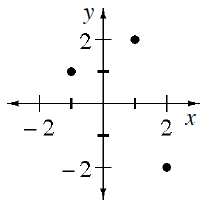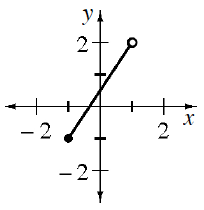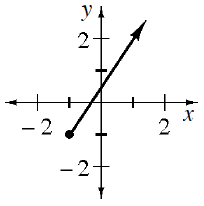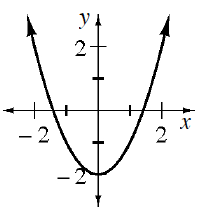### Home > INT3 > Chapter Ch1 > Lesson 1.1.4 > Problem1-61

1-61.

Name the domain and range for each of the following functions.

1.Range:
$\left\{−2,1,2\right\}$

Domain:
$\left\{−1,1,2\right\}$

1.Domain:
$−1\le x<1$

Range:
$−1\le y<2$

1.Domain:
$x\ge−1$

Range:
$y\ge−1$

1.Range:
$y\ge−2$

Domain:
$−∞